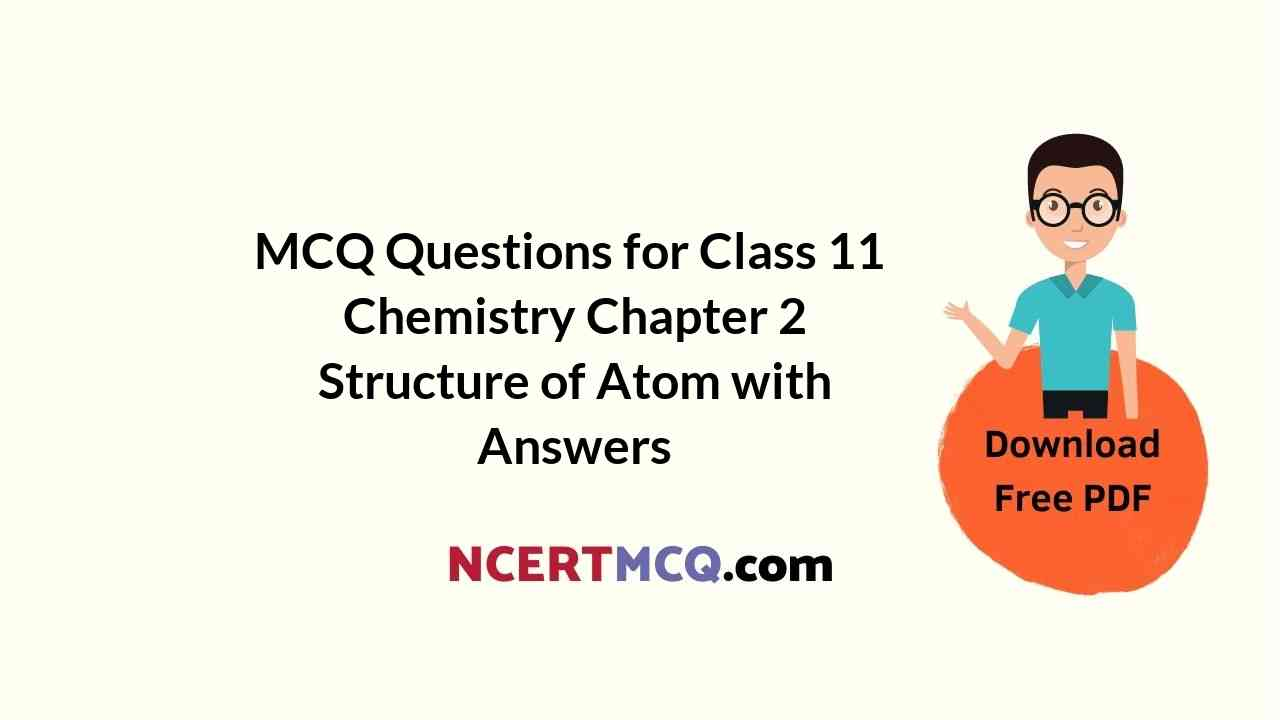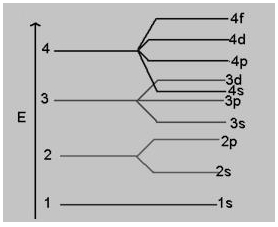Check the below NCERT MCQ Questions for Class 11 Chemistry Chapter 2 Structure of Atom with Answers Pdf free download. MCQ Questions for Class 11 Chemistry with Answers were prepared based on the latest exam pattern. We have provided Structure of Atom Class 11 Chemistry MCQs Questions with Answers to help students understand the concept very well.

## Structure of Atom Class 11 MCQs Questions with Answers

Structure Of Atom Class 11 MCQ Question 1.
The increasing order (lowest first) for the values of e/m (charge/mass) for
(a) e, p, n, α
(b) n, p, e, α
(c) n, p, α, e
(d) n, α, p, e

Answer: (d) n, α, p, e
Explanation:
(i) (e/m) for (i) neutron = ($$\frac{0}{1}$$) = 0
(ii) α− particle = ($$\frac{2}{4}$$) = 0.5
(iii) Proton = ($$\frac{1}{1}$$) = 1
(iv) electron = ($$\frac{1}{1837}$$) = 1837.

Structure Of Atom MCQ Class 11 Question 2.
The ionization enthalpy of hydrogen atom is 1.312 × 106 J mol-1. The energy required to excite the electron in the atom from n = 1 to n = 2 is
(a) 8.51 × 105 J mol-1
(b) 6.56 × 105 J mol-1
(c) 7.56 × 105 J mol-1
(d) 9.84 × 105 J mol-1

Answer: (d) 9.84 × 105 J mol-1
Explanation:
Energy required when an electron makes transition from n = 1 to n = 2
E2=−(1.312 × 106 × (1)²)/(2²)
= −3.28 × 105 J mol-1
E1 = −1.312 × 106 J mol-1
ΔE = E2 − E1
=−3.28 × 105−(−13.2 × 106)
ΔE = 9.84×105 J mol-1

Class 11 Chemistry Chapter 2 MCQ Question 3.
or a given principal level n = 4, the energy of its subshells is in the order
(a) s < p < d < f
(b) s > p > d > f
(c) s < p < f < d
(d) f < p < d < s

Answer: (a) s < p < d < f
Explanation:
Order of energy is:
s < p < d < fMCQ Questions For Class 11 Chemistry Chapter 2 Question 4.
A gas absorbs a photon of 355 nm and emits at two wavelengths. If one of the emissions is at 680 nm, the other is at:
(a) 518 nm
(b) 1035 nm
(c) 325 nm
(d) 743 nm

Explanation:
From Law of Conservation of energy, energy of absorbed photon must be equal to combined energy of two emitted photons.
ET = E1 + E2 ….. (1)
Where E1 is Energy of first emitted photon emitted and E2is Energy of second emitted photon.
Energy E and wavelength λ of a photon are related by the equation
E= (hc)/ (λ)….. (2)
Where Plancks constant = h, c is velocity of light.
Substituting the values from (2) in (1) we get
(hc/λT) = (hc)/ (λ1) + (hc)/ (λ2)
Or ($$\frac{1}{λ_T}$$) = ($$\frac{1}{λ_1}$$) + ($$\frac{1}{λ_2}$$) …… (3)
Substituting given values in (3) we get
($$\frac{1}{355}$$) = ($$\frac{1}{680}$$) + ($$\frac{1}{λ_2}$$)
Or $$\frac{(1)}{(λ_T)}$$ = ($$\frac{1}{355}$$) − ($$\frac{1}{680}$$)
⇒ ($$\frac{1}{λ_2}$$) = (680 − 355)/ (355 × 680)
⇒ λ2 = 742.77nm

Class 11 Chemistry Chapter 2 MCQ With Answers Question 5.
Which of the following statements in relation to the hydrogen atom is correct?
(a) 3s orbital is lower in energy than 3p orbital
(b) 3p orbital is lower in energy than 3d orbital
(c) 3s and 3p orbitals are of lower energy than 3d orbital
(d) 3s, 3p and 3d orbitals all have the same energy

Answer: (d) 3s, 3p and 3d orbitals all have the same energy
Explanation:
A hydrogen atom has 1st configuration and these its, 3p and 3d orbitals will have same energy wrt 1s orbital.

MCQ Of Structure Of Atom Class 11 Question 6.
The magnetic quantum number specifies
(a) Size of orbitals
(b) Shape of orbitals
(c) Orientation of orbitals
(d) Nuclear Stability

Explanation:
The magnetic quantum number specifies orientation of orbitals.

MCQ On Structure Of Atom Class 11 Question 7.
The electronic configuration of silver atom in ground state is
(a) [Kr]3d104s1
(b) [Xe]4f145d106s1
(c) [Kr]4d105s1
(d) [Kr]4d95s2

Explanation:
The electronic configuration of Ag in ground state is [Kr]4d105s1

Ch 2 Chemistry Class 11 MCQ Question 8.
Which of the following element has least number of electrons in its M-shell?
(a) K
(b) Mn
(c) Ni
(d) Sc

Explanation:
K = 19 = 1s²2s22p63s23p6s1
3s23p6 = m-shell
= k has only 8 electrons in M shell

Structure Of Atom Class 11 MCQ Questions And Answers Pdf Question 9.
Which one of the following sets of ions represents a collection of isoelectronic species? (Atomic nos.: F = 9, Cl = 17, Na = 11, Mg = 12, Al = 13, K = 19, Ca = 20, Sc = 21)
(a) K+, Ca2+, Sc3+, Cl
(b) Na+, Ca2+ , Sc3+, F
(c) K+, Cl, Mg2+, Sc3+
(d) Na+, Mg2+, Al3+, Cl

Answer: (a) K+, Ca2+, Sc3+, Cl
Explanation:
Isoelectronic species are those which have same number of electrons.
K+ = 19 – 1 = 18; Ca2+ = 20 – 2 = 18; Sc3+ = 21 – 3 = 18; Cl = 17 + 1 = 18
Thus all these ions have 18 electrons in them.

Atomic Structure Class 11 MCQ Questions Question 10.
In the ground state, an element has 13 electrons in its M-shell. The element is_____.
(a) Copper
(b) Chromium
(c) Nickel
(d) Iron

Explanation:
M shell means it is third shell ⇒ n = 3
Number of electrons in M shell = 13
⇒ 3s23p63d5
The electronic configuration is: (1s2) (2s2 2p6) (3s2 3p6 3d5) (4s1)
The element is chromium is Cr.

Chapter 2 Chemistry Class 11 MCQ Question 11.
The electrons of the same orbitals can be distinguished by
(a) Principal quantum number
(b) Azimuthal quantum number
(c) Spin quantum number
(d) Magnetic quantum number

Explanation:
Electrons occupying the same orbital are distinguished by Spin quantum number.
For spin Quantum number it has two values +1/2 or -1/2,
Hence the value of n, l , m are same for the two electrons occupying in the same orbitals, but only the is different, which is
Therefore, Spin quantum number explains the direction through which the electron spins in an orbital. so obviously there are only 2 possible directions. Which is either clockwise or anticlockwise.
So the electron which are available in the same orbitals, must have opposite spins. Hence spin quantum number distinguished b/w the two electrons.

Class 11 Chemistry Ch 2 MCQ Question 12.
Consider the ground state of Cr atom (Z = 24). The numbers of electrons with the azimuthal quantum numbers, l = 1 and 2 are, respectively:
(a) 12 and 4
(b) 12 and 5
(c) 16 and 4
(d) 16 and 5

Explanation:
24Cr → 1s2 2s22p6 3s2 3p6 3d5 4s1
As we know for p, l = 1 and d, l = 2
For l = 1, total number of electrons = 12 [2p6 and 3p6]
For l = 2, total number of electrons = 5 [3d5]

Chemistry Class 11 Chapter 2 MCQ Question 13.
A body of mass 10 mg is moving with a velocity of 100 ms-1. The wavelength of de-Broglie wave associated with it would be (Note: h = 6.63 × 10-34 Js)
(a) 6.63 × 10-37 m
(b) 6.63 × 10-31 m
(c) 6.63 × 10-34 m
(d) 6.63 × 10-35 m

Answer: (b) 6.63 × 10-31 m
Explanation:
m = 10 mg
= 10 × 10-6 kg
v = 100 ms-1
λ = $$\frac {(h)}{(mv)}$$
= (6.63×10-34)/ (10 × 10-6 × 100)
= 6.63 × 10-31 m

MCQ Of Chapter 2 Chemistry Class 11 Question 14.
The ionization enthalpy of hydrogen atom is 1.312 × 106 J mol-1. The energy required to excite the electron in the atom from n = 1 to n = 2 is
(a) 8.51 × 105 J mol-1
(b) 6.56 × 105 J mol-1
(c) 7.56 × 105 J mol-1
(d) 9.84 × 105 J mol-1

Answer: (d) 9.84 × 105 J mol-1
Explanation:
Energy required when an electron makes transition from n = 1 to n = 2
E2 = −(1.312 × 106 × (1)²)/(2²)
= −3.28 × 105 J mol-1
E1 = −1.312 × 106 J mol-1
ΔE = E2 − E1
= −3.28 × 105−(−13.2 × 106)
ΔE = 9.84 × 105 J mol-1

Class 11 Chemistry Chapter 2 MCQ Questions With Answers Question 15.
In Hydrogen atom, energy of first excited state is – 3.4 eV. Then find out KE of same orbit of Hydrogen atom
(a) 3.4 eV
(b) 6.8 eV
(c) -13.6 eV
(d) +13.6 eV

Explanation:
For hydrogen atom,
The kinetic energy is equal to the negative of the total energy.
And the potential energy is equal to the twice of the total energy.
The first excited state energy of orbital = -3.4 eV
and The kinetic energy of same orbital = -(-3.4 eV) = 3.4 eV
Therefore, the kinetic energy of same orbit of hydrogen atom is 3.4 eV.

Question 16.
Which of the following sets of quantum numbers represents the highest energy of an atom?
(a) n = 3, l = 0, m = 0, s = + $$\frac {1}{2}$$
(b) n = 3, l = 1, m = 1, s = + $$\frac {1}{2}$$
(c) n = 3, l = 2, m = 1, s = + $$\frac {1}{2}$$
(d) n = 4, l = 0, m = 0, s = + $$\frac {1}{2}$$

Answer: (c) n = 3, l = 2, m = 1, s = + $$\frac {1}{2}$$
Explanation:
n = 3, l = 0 represents 3s orbital n = 3, l = 1 represents 3p orbital n = 3, l = 2 represents 3d orbital n = 4, l = 0 represents 4s orbital The order of increasing energy of the orbitals is 3s < 3p < 4s < 3d.

Question 17.
In the Bohrs model of the hydrogen atom, the ratio of the kinetic energy to the total energy of the electron in a quantum state n is:
(a) 1
(b) 2
(c) -1
(d) -2

Explanation:
As we know, in Bohr model KE of an electron in an orbit = + ($$\frac {1}{2}$$) ($$\frac {e^2}{r_n}$$)
Total energy of electron in an orbit = -($$\frac {e^2}{2r_n}$$)
Therefore, $$\frac {(KE)}{(TE)}$$ = -1

Question 18.
Which of the following statements does not form a part of Bohrs model of hydrogen atom?
(a) Energy of the electrons in the orbit is quantised
(b) The electron in the orbit nearest the nucleus has the lowest energy
(c) Electrons revolve in different orbits around the nucleus
(d) The position and velocity of the electrons in the orbit cannot be determined simultaneously

Answer: (d) The position and velocity of the electrons in the orbit cannot be determined simultaneously
Explanation:
The position and velocity of electrons cannot be determined simultaneously does not fit in with the Bohrs model of H atom. It is a part of Heisenbergs uncertainty principle

Question 19.
Which of the following statements in relation to the hydrogen atom is correct?
(a) 3s orbital is lower in energy than 3p orbital
(b) 3p orbital is lower in energy than 3d orbital
(c) 3s and 3p orbitals are of lower energy than 3d orbital
(d) 3s, 3p and 3d orbitals all have the same energy

Answer: (d) 3s, 3p and 3d orbitals all have the same energy
Explanation:
A hydrogen atom has 1s1 configuration and these its, 3p and 3d orbitals will have same energy wrt 1s orbital.

Question 20.
A sub-shell with n = 6 , l = 2 can accommodate a maximum of
(a) 12 electrons
(b) 36 electrons
(c) 10 electrons
(d) 72 electrons